## Feasibility Study Using Excel

To put all previous cost and benefit formulas of Bus feasibility study in MS Excel, we better separate input of user (constants) from the formulas and result of computation. You can see the relationship between all formulas in the diagram below. This diagram is useful to separate them.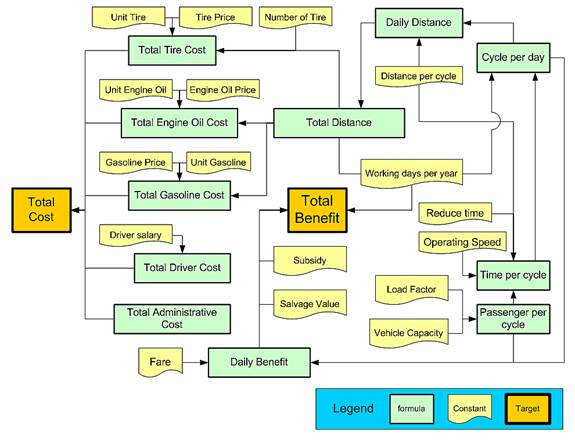Since we have two type of vehicles, we put them in two columns so that easier to copy. MS Excel file companion of this tutorial can be downloaded here .

The formulas are put in separate places so that we obtain all results in one area of the spreadsheet.

Several notes must be given regarding the formulas:

1. All formulas for operation, benefit, cost and evaluation above are based on equation (1) to (14) above.
2. Annual Salvage value are converted from Future Value into Annuity using either MS Excel function =-PMT (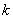,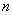,0,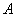) or multiply with factor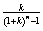3. Present Total Cost,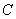, consist of initial cost and converted value of total operating cost. The conversion is using MS Excel function =-PV (,,) or multiply with factor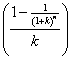. Then we put negative sign to this formula to make it negative value. This is useful step to calculate the IRR using MS Excel function.
4. Present Total Benefit is converted from the summation of annual operating benefit plus subsidy and annual salvage value. The conversion factor is similar to Present Total Cost without the negative sign.
5. NPV and BCR should consider the negative sign of Present Total Cost
6. IRR is using MS Excel function =IRR(array of cost and benefit)

he results of this simple computation reveal that both minibus and minivan are both have positive NPV (BCR>1 and IRR> interest rate). Thus both vehicle types are feasible. They can be operated without any local government subsidy. This will be good news for the local government who hire you as consultant. They may hire you again next time for better and larger project. Of course, among the two types of proposed buses, minibus is the most feasible.

Preferable reference for this tutorial is

Teknomo, Kardi (2006) Tutorial on Feasibility Study. http://people.revoledu.com/kardi/tutorial/What-If-Analysis/index.html